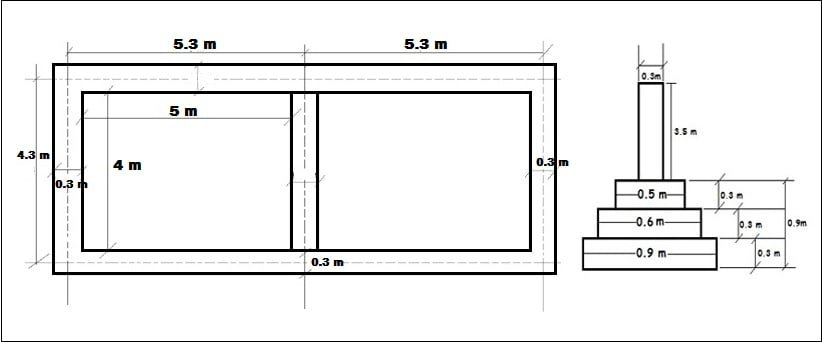## Register Now

Lorem ipsum dolor sit amet, consectetur adipiscing elit.Morbi adipiscing gravdio, sit amet suscipit risus ultrices eu.Fusce viverra neque at purus laoreet consequa.Vivamus vulputate posuere nisl quis consequat.

## Center Line Method Of Estimation:

There are three different methods of estimation.

1. Center line method.
2. Long and short wall method.
3. Crossing method.

In this article, I will discuss center line method briefly for the estimation of materials.

## What Is Center Line Method:

In this method of estimation, the total center line length of walls in a building is first calculated, then the center line length is multiplied with the breadth and depth of respective item to get the total quantity at a time.

The center line length for different sections of walls in a building shall be worked out separately. For verandah walls or partition walls joining the main walls, the center line length shall be reduced by half of the breadth of the layer of main wall that joins with the partition or verandah wall at the same level. The number of such joints is studied first to obtain the center line length.

By using this method estimation can be finished more quickly. This method is as accurate as other methods (except unsymmetrical wall). This method is suitably used for estimating circular, rectangular, hexagonal, octagonal etc shaped building.

Let us take an example:

## For One Room Building:First we need to calculate total center line length of the building.

Total center line length = 2 x 5.3 + 2 x 4.3 = 19.2 m.

1. Earthwork in excavation = Total center line length x breadth x depth

= 19.2 x 0.9 x (0.3+0.3+0.3)

= 19.2 x 0.9 x 0.9

= 15.52 cu.m

2. Concrete in foundation = 19.2 x 0.9 x 0.3 = 5.18 cu.m

3. a) Brickwork in foundation for 1st footing = 19.2 x 0.6 x 0.3 cu.m

b) Brickwork in foundation for 2nd footing = 19.2 x 0.5 x 0.3 = 2.88 cu.m

4. Brickwork in superstructure = 19.2 x 3.5 x 0.3 = 20.16 cu.m

## For Two Room Building:Total center line length = 2 x (5.3+5.3) + 3 x 4.3 = 34.1 m.

1. Earthwork in excavation = Total center line length x breadth x depth

= 34.1 x 0.9 x (0.3+0.3+0.3)

= 34.1 x 0.9 x 0.9

= 27.62 cu.m

2. Concrete in foundation = 34.1 x 0.9 x 0.3 = 9.20 cu.m

3. a) Brickwork in foundation for 1st footing = 34.1 x 0.6 x 0.3 = 6.13 cu.m

b) Brickwork in foundation for 2nd footing = 34.1 x 0.5 x 0.3 = 5.11 cu.m

4. Brickwork in superstructure = 34.1 x 3.5 x 0.3 = 35.8 cu.m

Thus you can estimate quantity of different items such as

Quantity of D.P.C = Total Centre line length x Breadth of foundation x Thickness of D.P.C

Quantity of plinth beam = Total Centre line length x Breadth of the beam x Depth of beam.

Quantity of wall plaster for 2 sides = Total centre line length x Height of wall x Thickness of plaster.

Quantity of Paint for 2 sides of wall = Total centre line length x Height of wall  x 2  = Area of paint in sq.ft

#### About Admin1. layout of buildind practical file in hindi 5by4
appretus……..?
method………?
result…………?
dising …….?

2. Very useful for beginners.

3. nice information

4. there should be two raw plan its quit nice information but i want to learn more then 5 room plan please provide if you can

5. What is main principle of center line method..?

6. Very useful information

7. It is good information

error: Content is protected !!